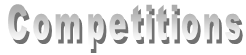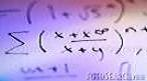APICS competition for undergraduate students The APICS Math competition is a mathematical problem solving competition written at the annual APICS Mathematics and Statistics meeting.Mathematical Contest in Modeling The Mathematical Contest in Modeling is an international contest administered by the Consortium on Mathematics and its Applications (CoMAP) and sponsored by CoMAP, the Mathematical Association of America (MAA), the Society of Industrial and Applied Mathematics (SIAM), the National Security Agency (NSA) and others. Each team of three students is given two real-world problems, one involving the mathematics of continuous change and the other involving discrete mathematics. The team picks a problem and works on it over an extended weekend. Their entry is a paper which applies mathematics to the problem and explains why they took their approach, how they tested their ideas and the limitations of their ideas. In 1999, a second contest, the Inter-disciplinary Contest in Modeling was introduced; the ICM does not offer a choice of problems, but the two contests are otherwise comparable.Putnam Competition The examination will be constructed to test originality as well as technical competence. It is expected that the contestant will be familiar with the formal theories embodied in undergraduate mathematics. It is assumed that such training, designed for mathematics and physical science majors, will include somewhat more sophisticated mathematical concepts than is the case in minimal courses. Thus the differential equations course is presumed to include some references to qualitative existence theorems and subtleties beyond the routine solution devices. Questions will be included that cut across the bounds of various disciplines, and self-contained questions that do not fit into any of the usual categories may be included. It will be assumed that the contestant has acquired a familiarity with the body of mathematical lore commonly discussed in mathematics clubs or in courses with such titles as “survey of the foundations of mathematics.” It is also expected that the self-contained questions involving elementary concepts from group theory, set theory, graph theory, lattice theory, number theory, and cardinal arithmetic will not be entirely foreign to the contestant’s experience.

Chase Building | Dalhousie University | Halifax, Nova Scotia, Canada B3H 3J5 | +1 (902) 494-2572